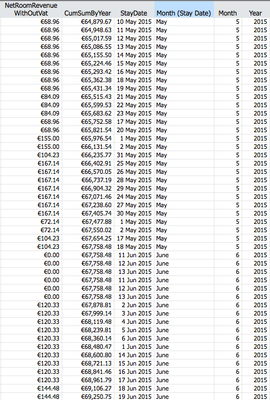Our World Statistics Day conversations have been a great reminder of how much statistics can inform our lives. Do you have an example of how statistics has made a difference in your life? Share your story with the Community!
Choose Language Hide Translation Bar
Highlighted

## Col (Sum) function by selecting specific rows

Hello,

I would like to create a column which calculates another columns mean or sum but I would like to specify the function by :

Let’s say if we select the year the calculation to be occured by "Year" or,

Let’s say col (sum) of NetRevenueWithoutVat by Year or "NetRevenueWithoutVat" by "Month (Stay Date)" or

Col (Sum) functions of specific row numbers...

Would anyone please share his/her knowledge how to do it in scripting or by entering the Col(Sum) function as a formula in a column?

The logic behind it?1 ACCEPTED SOLUTION

Accepted Solutions
Highlighted

## Re: Col (Sum) function by selecting specific rows

Use ColSum() in column formulas. The formula Col Sum( :NetRevenueWithoutVat, :Year ) will return the sum (not the cumulative sum) grouped by Year.

Try this formula for a ColSum() that changes dynamically with row selection:

Col Sum( :NetRevenueWithoutVat, Selected() )

16 REPLIES 16
Highlighted

## Re: Col (Sum) function by selecting specific rows

The answer may depend on what happens next. You can certainly do:

``````NamesDefaultToHere(1);
// (1) Get some data
dt1 = Open("\$SAMPLE_DATA/Big Class.jmp");
// (2) Get summary info into a list and a vector
Summarize(groups = By(:sex), groupMeans = Mean(:height));
// (3) Make a table using the summary info
dt2 = NewTable("Height by Gender",
NewColumn("Gender", Character, Nominal, Values(groups)),
NewColumn("Mean Height", Numeric, Continuos, Values(groupMeans))
);``````

(see the JSL Scripting Guide for more options with Summarise).

If you need step 3, you may be better using either 'Tables > Summary' or 'Analyse > Tabulate'.

You can also aggregate across any set of rows, so long as you can express what that selection is.

Highlighted

## Re: Col (Sum) function by selecting specific rows

Use ColSum() in column formulas. The formula Col Sum( :NetRevenueWithoutVat, :Year ) will return the sum (not the cumulative sum) grouped by Year.

Try this formula for a ColSum() that changes dynamically with row selection:

Col Sum( :NetRevenueWithoutVat, Selected() )

Highlighted

## Re: Col (Sum) function by selecting specific rows

Thank you!

This is what I was looking for.

Does that mean I could go further and let's say Col Sum( :NetRevenueWithoutVat, :Year, :Agency )  ?

Highlighted

## Re: Col (Sum) function by selecting specific rows

Yes, more than one grouping variable is supported.

Highlighted

## Re: Col (Sum) function by selecting specific rows

Are you able to use this formula and define the logic for a particular column? For example, I am trying to sum the dollar amounts for each record between two dates. So the formula would look like this:

Col Sum( :Dollar Amount, :Date)

But I am not sure how to define the date range.

Highlighted

## Re: Col (Sum) function by selecting specific rows

@Mitch103
Try something like this as suggested by @ms

``````dt = Current Data Table();

dt << Select Where(:Date > StartDate & :Date <EndDate);

dt << New Column("ConditionalSum",Numeric,continuous,Formula(Col Sum(:Date,Selected())); ``````
Best
Uday
Highlighted

## Re: Col (Sum) function by selecting specific rows

So forgive me, but I am a new JMP user. I am only using the formula editor, not JSL. I am not sure if what you are giving me is for JSL or the forumula editor. Am I not able to put the conditions into the formula like this?

Col Sum(:Settlement Amount,:Date >= 8/1/2018 & :Date <= 8/7/2018,:Card Number)

Except the problem I am running into is that JMP is autocorrecting it to be division, not a date formula.

Highlighted

## Re: Col (Sum) function by selecting specific rows

Nevermind, got it!

Col Sum(
:Settlement Amount,
:Date >= 01Aug2018 & :Date <= 07Aug2018,
:Card Number
)
Highlighted

## Re: Col (Sum) function by selecting specific rows

You would have to create a separate column that contains a grouping column for each date range you want to use, or you might want to use something like:

``````Col Sum(
If(
:Event Date >= Informat( "02/01/2001", "mm/dd/yyyy" ) & :Event Date <=
Informat( "02/28/2001", "mm/dd/yyyy" ),
:Total Fatal Injuries,
0
)
)``````
Jim
Article Labels

There are no labels assigned to this post.